# C++ 学习笔记（一）：初学者的小窍门

## C++ Study Notes (1) : Tips for beginner

Thistledown    February 27, 2019

## C++ 数据类型及其表示范围

#### 1. 基本的内置类型

C++ 为程序员提供了种类丰富的内置数据类型和用户自定义的数据类型。下表列出了七种基本的 C++ 数据类型：

1
typedef wchar_t short int;


• signed
• unsigned
• short
• long

#### 2. 各种变量类型的大小

char 1 $-128 \sim 127 \ ||\ 0 \sim 255$
unsigned char 1 $0 \sim 255$
signed char 1 $-128 \sim 127$
int 4 $-2^{31} \sim 2^{31}-1$
unsigned int 4 $0 \sim 2^{32} - 1$
signed int 4 $-2^{31} \sim 2^{31}-1$
short int 2 $-2^{15} \sim 2^{15}-1$
unsigned short int 2 $0 \sim 2^{16} - 1$
signed short int 2 $-2^{15} \sim 2^{15}-1$
long int 8 $-2^{63} \sim 2^{63} - 1$
unsigned long int 8 $0 \sim 2^{64} - 1$
signed long int 8 $-2^{63} \sim 2^{63} - 1$
float 4 $-3.40 \times 10^{38} \sim 3.40 \times 10^{38}$
double 8 $-1.79\times10^{308} \sim 1.79 \times 10^{308}$

## 浮点数的比较

1
const double eps = 1e-8;


#### 1. 等于运算符 ==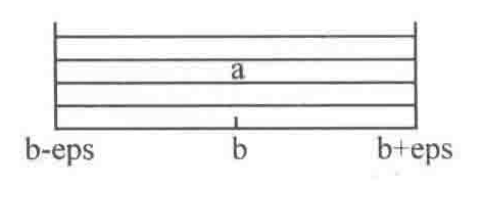1
2
#include <math.h>
#define Equ(a, b) ((fabs((a) - (b))) - (eps)) // 括号是为防止宏定义可能带来的错误


#### 2. 大于运算符 >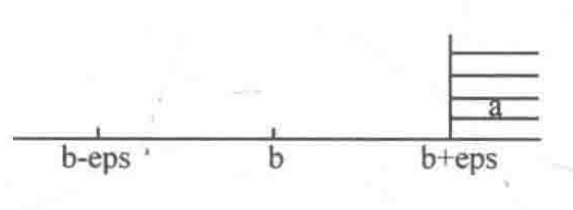1
#define More(a, b) (((a) - (b)) > (eps))


#### 3. 小于运算符 <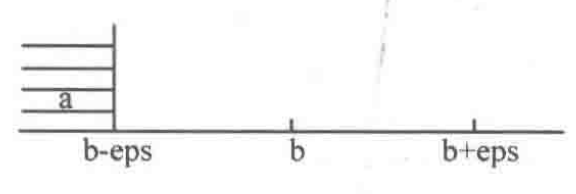1
#define Less(a, b) (((a) - (b)) < (-eps))


#### 4. 大于等于运算符 >=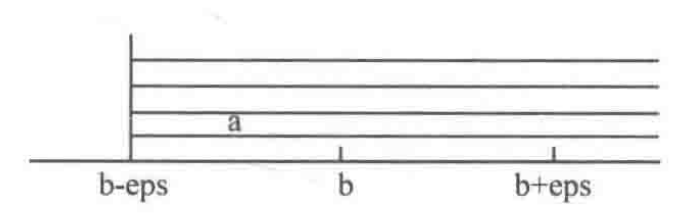1
#define MoreEqu(a, b) (((a) - (b)) > (-eps))


#### 5. 小于等于运算符 <=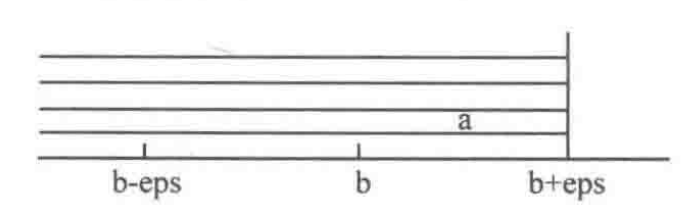1
#define LessEqu(a, b) (((a) - (b)) < (eps))


#### 6. 圆周率 π

1
2
#include <math.h>
const double PI = acos(-1.0);


1
2
3
4
5
6
7
8
9
#include <math.h>
const double eps = 1e-8;
const double PI = acos(-1.0);

#define Equ(a, b) ((fabs((a) - (b))) - (eps))
#define More(a, b) (((a) - (b)) > (eps))
#define Less(a, b) (((a) - (b)) < (-eps))
#define MoreEqu(a, b) (((a) - (b)) > (-eps))
#define LessEqu(a, b) (((a) - (b)) < (eps))


## 读取“大数”

C 库函数 int getchar(void) 从标准输入 stdin 获取一个字符（一个无符号字符）。这等同于 getc 带有 stdin 作为参数。

1
2
3
4
5
6
7
8
9
10
11
12
13
14
15
16
17
18
#include <stdio.h>

int main() {
char n;
int i = 0;
printf("Input:  ");
while ((n[i] = getchar()) != '\n') {    // 逐位读入数字
i++;
}

printf("Output: ");
for (int k = 0; k < i; k++) {
printf("%c", n[k]);     // 逐位输出数字
}

printf("\n\n");
return 0;
}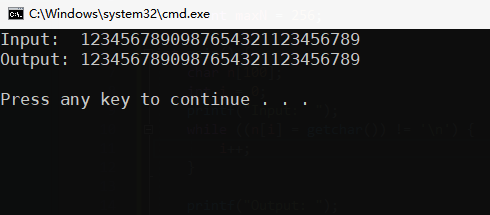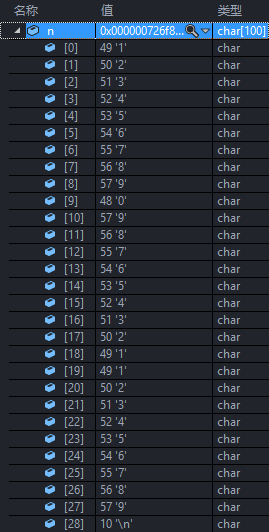Thistledown